Like   Tweet   Pin   +1   in
 /* styles */
 table div table+table+table div table{width:100%;padding:0}table div table+table+table div table img{width:96.23%;padding:0;float:none}table div table+table+table div table td{width:100%;padding:0 1.88% 18px}/* styles */## Member and Faculty News

 table div table+table+table+table+table+table div table{width:100%;padding:0}table div table+table+table+table+table+table div table img{width:96.23%;padding:0;float:none}table div table+table+table+table+table+table div table td{width:100%;padding:0 1.88% 18px}/* styles */## Highlights from the Summer

 table div table+table+table+table+table+table+table+table div table{width:100%;padding:0}table div table+table+table+table+table+table+table+table div table img{width:96.23%;padding:0;float:none}table div table+table+table+table+table+table+table+table div table td{width:100%;padding:0 1.88% 18px}/* styles */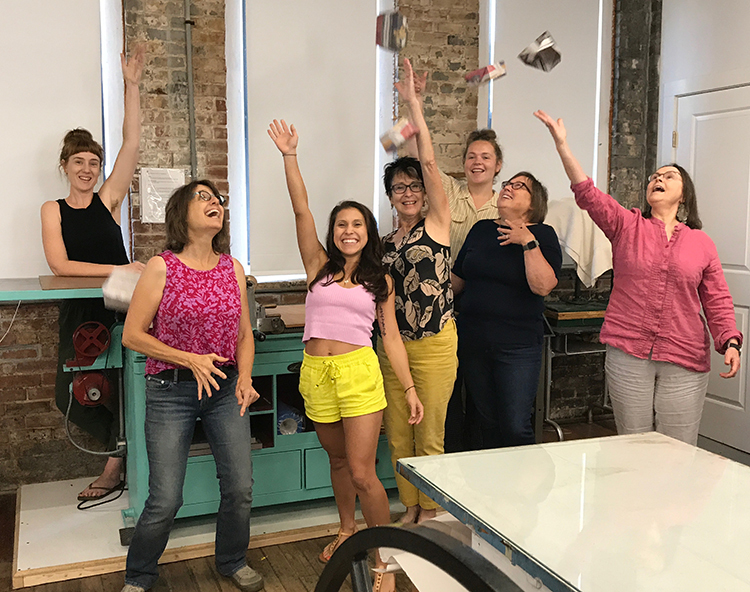7 of the 8 graduates of GPC19

# Green Printmaking Certificate: Intaglio 2019

We graduated another wonderful group of artists from our intensive etching program this summer.

They learned how to set up and maintain an etching studio, all kinds of intaglio processes, professional printing practices, and much more! Happy to send these now experienced etchers out into the world to spread the word of safer etching!

 table div table+table+table+table+table+table+table+table+table+table div table{width:100%;padding:0}table div table+table+table+table+table+table+table+table+table+table div table img{width:96.23%;padding:0;float:none}table div table+table+table+table+table+table+table+table+table+table div table td{width:100%;padding:0 1.88% 18px}/* styles */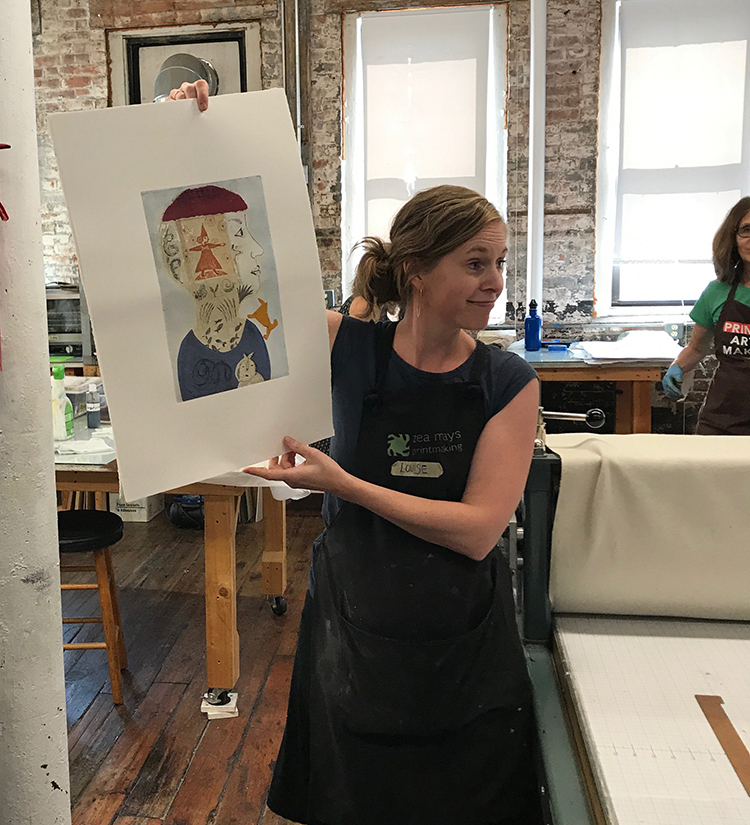Louise showing off Nancy Doniger's multi-plate w/ chine colle etching

# Other Summer intensives

Summer is the time for deep dives into a variety of printmaking experiences here at Zea Mays. Our summer line-up included

▪ an Advanced Etching workshop, where participants worked on multi-plate, color etchings
▪ A monotype intensive that included all kinds of mark making strategies
▪ A thorough investigation of photopolymer intaglio including manipulating films and plates
▪ And a week of Japanese Water-based Woodblock, where participants worked on up to 6 blocks.
 ▪ an Advanced Etching workshop, where participants worked on multi-plate, color etchings
 ▪ A monotype intensive that included all kinds of mark making strategies
 ▪ A thorough investigation of photopolymer intaglio including manipulating films and plates
 ▪ And a week of Japanese Water-based Woodblock, where participants worked on up to 6 blocks.
 table div table+table+table+table+table+table+table+table+table+table+table+table div table{width:100%;padding:0}table div table+table+table+table+table+table+table+table+table+table+table+table div table img{width:96.23%;padding:0;float:none}table div table+table+table+table+table+table+table+table+table+table+table+table div table td{width:100%;padding:0 1.88% 18px}/* styles */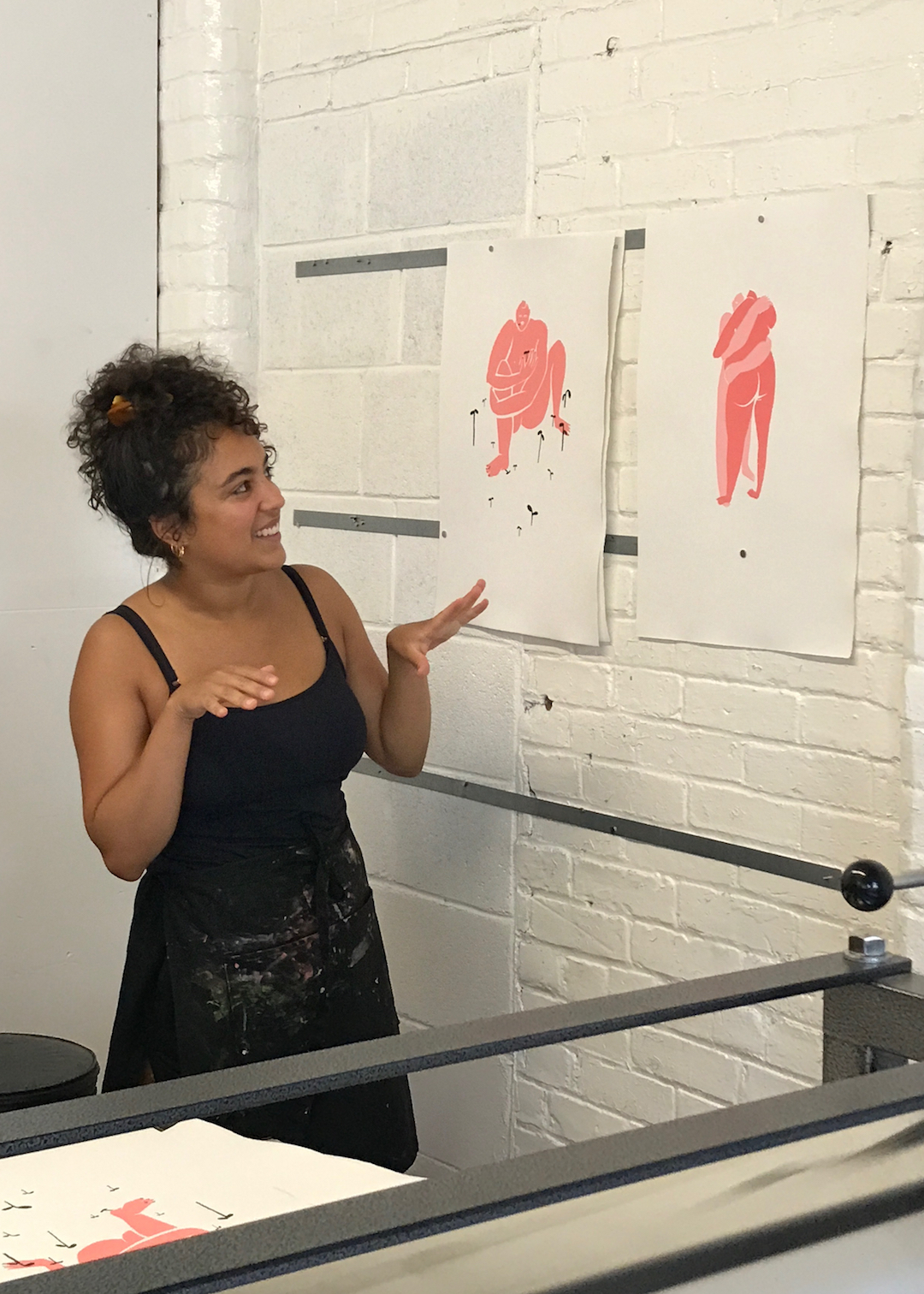Yasmina Malmsten presenting her work

# Summer Artists in Residence

We were pleased to welcome new and returning artists for residencies this summer. They always bring a shot of energy and excitement to the studio. This year's group included:
Jennifer Scheuer
Robin Gibson
Yasmina Malmstem
Lilla Szekely
Fran Valesco
Maggie Oakes
Lynn Manos

We're accepting applications for 2020 and 2021 residencies. The spots fill quickly, so apply early!

 table div table+table+table+table+table+table+table+table+table+table+table+table+table+table div table{width:100%;padding:0}table div table+table+table+table+table+table+table+table+table+table+table+table+table+table div table img{width:96.23%;padding:0;float:none}table div table+table+table+table+table+table+table+table+table+table+table+table+table+table div table td{width:100%;padding:0 1.88% 18px}/* styles */## What's Happening Now

 table div table+table+table+table+table+table+table+table+table+table+table+table+table+table+table+table div table{width:100%;padding:0}table div table+table+table+table+table+table+table+table+table+table+table+table+table+table+table+table div table img{width:96.23%;padding:0;float:none}table div table+table+table+table+table+table+table+table+table+table+table+table+table+table+table+table div table td{width:100%;padding:0 1.88% 18px}/* styles */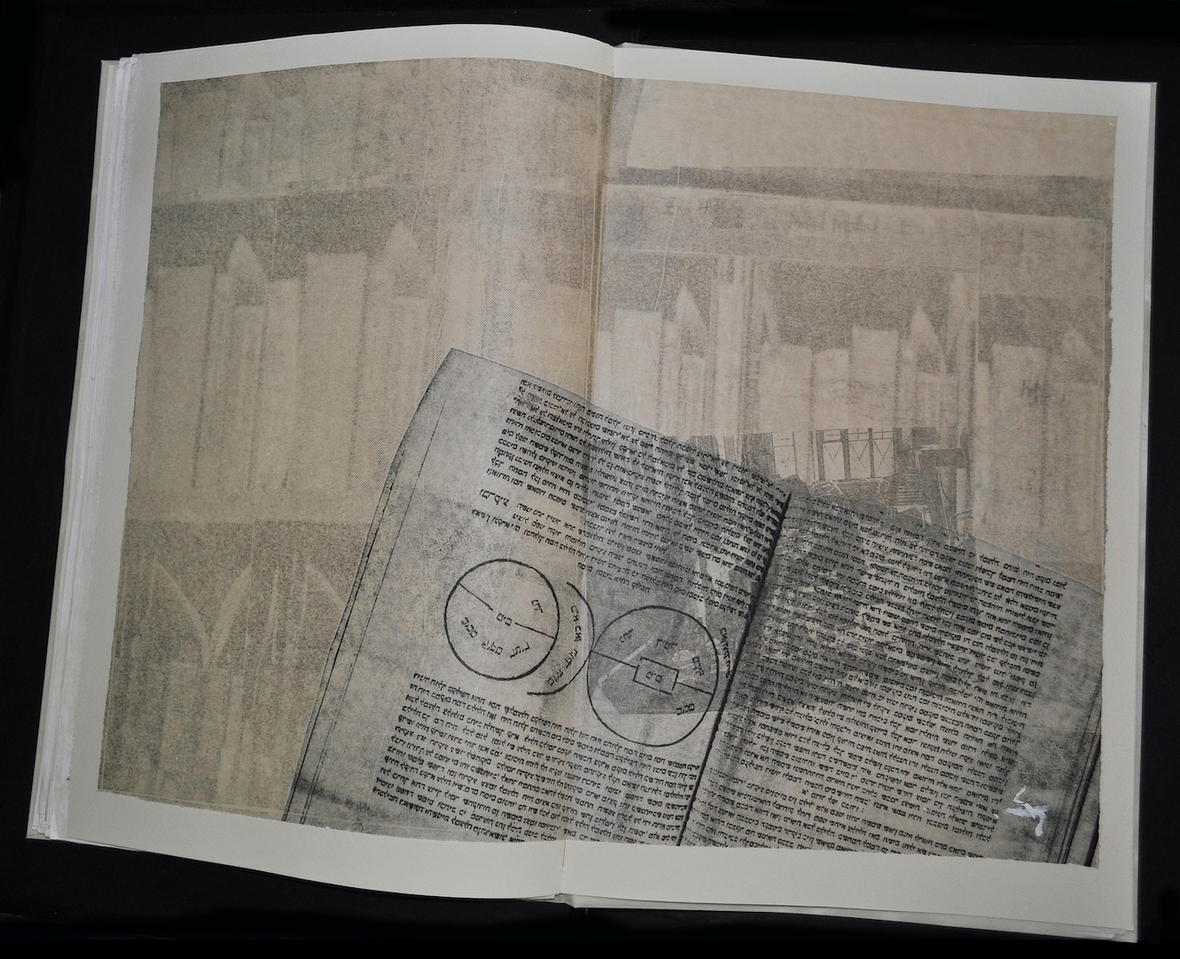Anne Beresford, "Via, Villa, Vista" artist's book

# In the Sanford Gallery @ ZMP

## Portfolios and Artists' Books

Featuring work by Anne Beresford, Liz Chalfin, Rachel Chapman, Amanda Maciuba, Tekla McInerney, Larinda Meade, Erika Radich, Edda Sigurdardottir, Vaune Trachtman, Louise Wallendorf, Esther S. White.

 table div table+table+table+table+table+table+table+table+table+table+table+table+table+table+table+table+table+table div table{width:100%;padding:0}table div table+table+table+table+table+table+table+table+table+table+table+table+table+table+table+table+table+table div table img{width:96.23%;padding:0;float:none}table div table+table+table+table+table+table+table+table+table+table+table+table+table+table+table+table+table+table div table td{width:100%;padding:0 1.88% 18px}/* styles */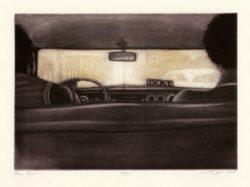Liz Chalfin, "Peso Taxi," etching

# Fall Workshop Registration

We have an exciting line-up of Fall Workshops - these still have spaces, but do fill quickly.

Etching Line and Tone (3 spots) September 28-29

Make an Impression (2 spots) October 5

Intaglio Meets Monotype (2 spots) October 19-20

Tuesday Morning Monotype: Play with the Plate October 22- November 19

Intro to Photopolymer Printmaking October 25-27

Artists' Books for Printmakers November 2-3

Layered Mixed Media Printmaking November 9-10

Your Art Better! November 15, December 11, 2019, February 12 and April 8, 2020

Drawing and Drypoint November 16-17

 table div table+table+table+table+table+table+table+table+table+table+table+table+table+table+table+table+table+table+table+table div table{width:100%;padding:0}table div table+table+table+table+table+table+table+table+table+table+table+table+table+table+table+table+table+table+table+table div table img{width:96.23%;padding:0;float:none}table div table+table+table+table+table+table+table+table+table+table+table+table+table+table+table+table+table+table+table+table div table td{width:100%;padding:0 1.88% 18px}/* styles */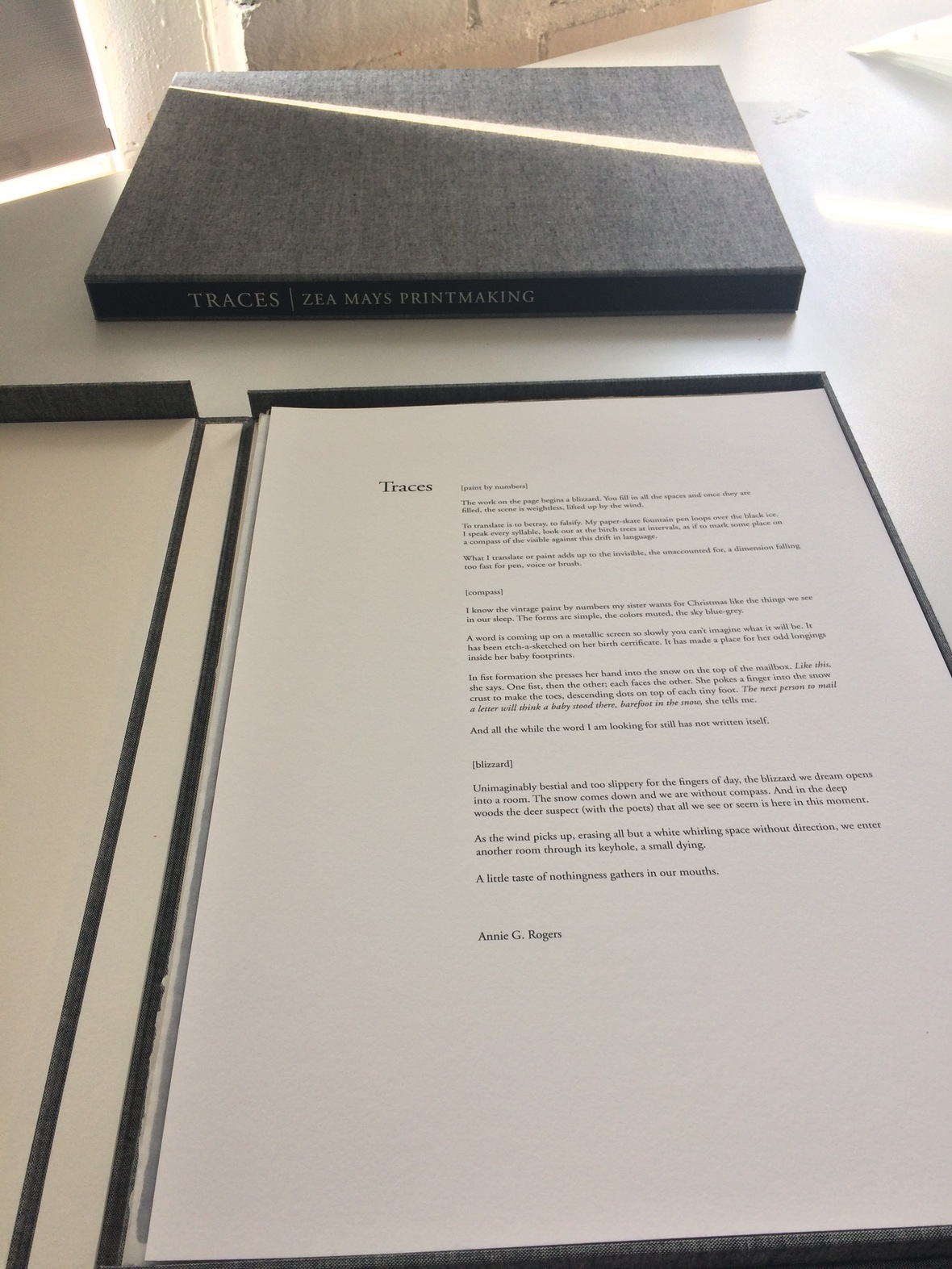# University Museum of Contemporary Art purchased "Traces" portfolio

We are thrilled to have our 2018 portfolio "Traces" in the collection of the UMCA at UMass, Amherst. It is extra meaningful to us that our work is part of the collection of a public university and belongs to all of the citizens of Massachusetts!

 table div table+table+table+table+table+table+table+table+table+table+table+table+table+table+table+table+table+table+table+table+table+table div table{width:100%;padding:0}table div table+table+table+table+table+table+table+table+table+table+table+table+table+table+table+table+table+table+table+table+table+table div table img{width:96.23%;padding:0;float:none}table div table+table+table+table+table+table+table+table+table+table+table+table+table+table+table+table+table+table+table+table+table+table div table td{width:100%;padding:0 1.88% 18px}/* styles */## What's to come

 table div table+table+table+table+table+table+table+table+table+table+table+table+table+table+table+table+table+table+table+table+table+table+table+table div table{width:100%;padding:0}table div table+table+table+table+table+table+table+table+table+table+table+table+table+table+table+table+table+table+table+table+table+table+table+table div table img{width:96.23%;padding:0;float:none}table div table+table+table+table+table+table+table+table+table+table+table+table+table+table+table+table+table+table+table+table+table+table+table+table div table td{width:100%;padding:0 1.88% 18px}/* styles */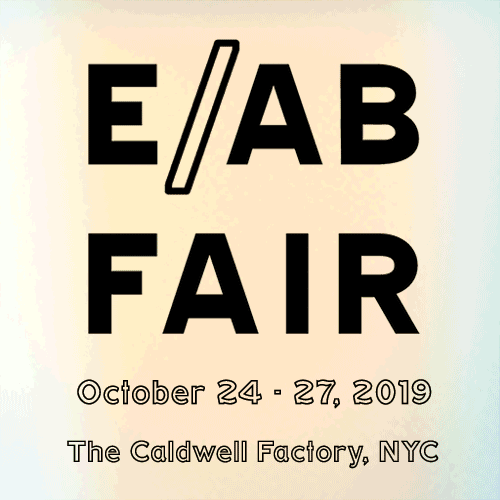# Editions/Artists' Book Fair

Zea Mays Printmaking will be returning to the legendary Editions/Artists' Book Fair for our fifth year. The venue has changed to The Caldwell Factory - a luxurious space in the heart of Chelsea. The fair will take place October 24 - 27, 2019

Zea Mays Printmaking's booth will be featuring the work of Anne Beresford, Annie Bissett, Liz Chalfin, T. Klacsmann, Kevin Pomerleau, Tekla McInerney, Erika Radich, Edda Sigurdardottir and Amanda Maciuba.

 table div table+table+table+table+table+table+table+table+table+table+table+table+table+table+table+table+table+table+table+table+table+table+table+table+table+table div table{width:100%;padding:0}table div table+table+table+table+table+table+table+table+table+table+table+table+table+table+table+table+table+table+table+table+table+table+table+table+table+table div table img{width:96.23%;padding:0;float:none}table div table+table+table+table+table+table+table+table+table+table+table+table+table+table+table+table+table+table+table+table+table+table+table+table+table+table div table td{width:100%;padding:0 1.88% 18px}/* styles */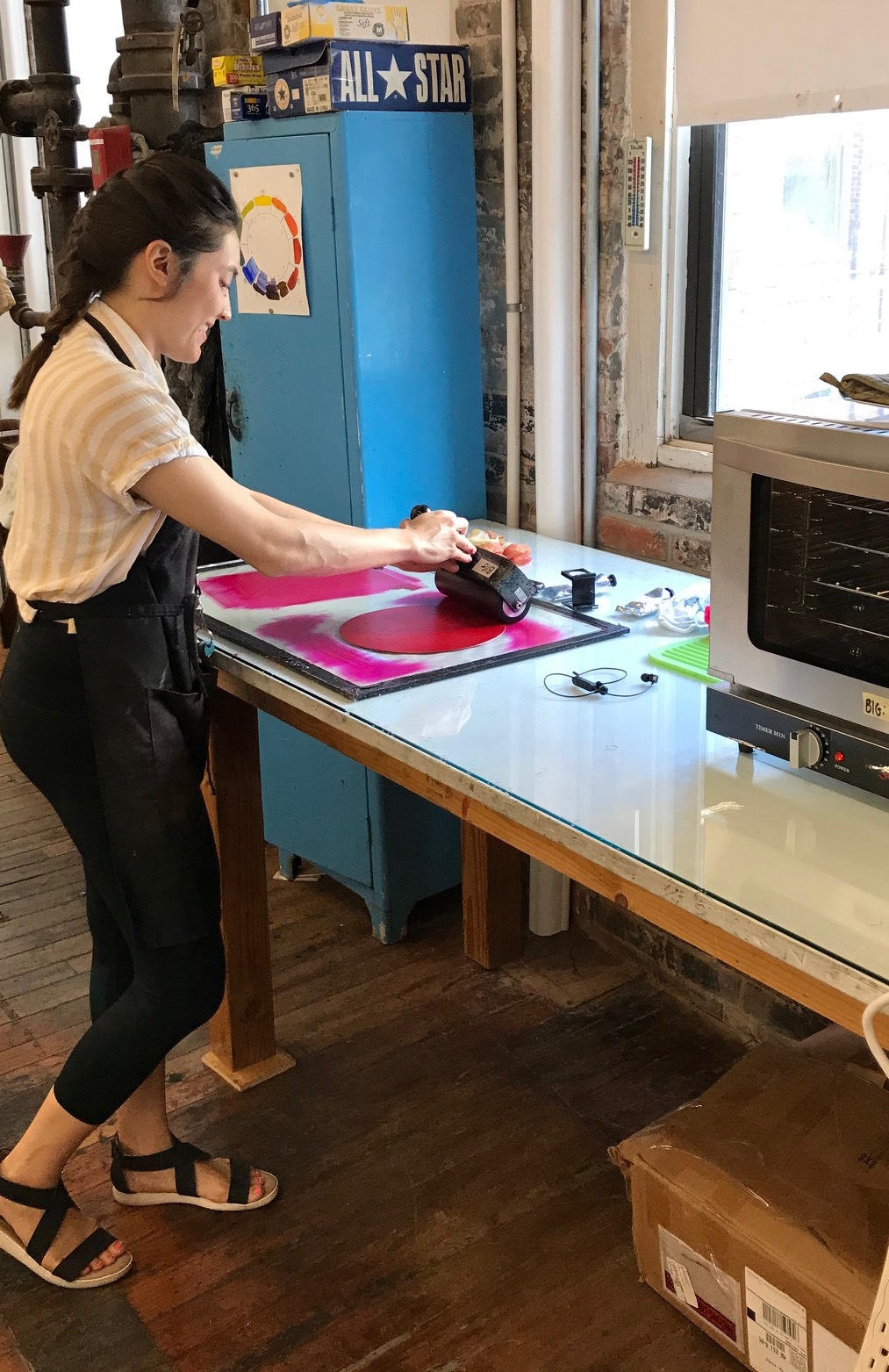2019 Artist in Residence, Jade Hoyer

# Artists in Residence

Our residency program is thriving with artists scheduled for the fall and winter of 2019 including:
angela hennessey
peter granucci
jennifer schmidt
cassandra van saun
laura asher
jean sanders

We're booking residencies for 2020 and beyond. Find information and application here.

 table div table+table+table+table+table+table+table+table+table+table+table+table+table+table+table+table+table+table+table+table+table+table+table+table+table+table+table+table div table{width:100%;padding:0}table div table+table+table+table+table+table+table+table+table+table+table+table+table+table+table+table+table+table+table+table+table+table+table+table+table+table+table+table div table img{width:96.23%;padding:0;float:none}table div table+table+table+table+table+table+table+table+table+table+table+table+table+table+table+table+table+table+table+table+table+table+table+table+table+table+table+table div table td{width:100%;padding:0 1.88% 18px}/* styles */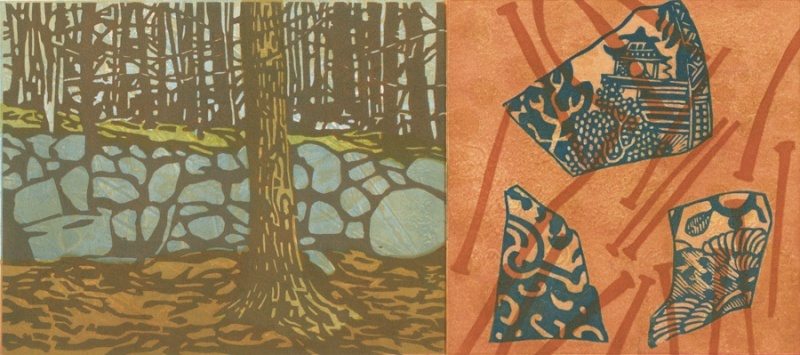Wendy Ketchum, "There are stories in These Walls of Stone," woodcut monoprint

# Autumn Light

## October 3-31

Although each season comes with its own beauty, autumn has a particular place in nature’s art. As summer slides into fall the change in the quality of the light is noticeable. The sun’s lower position in the sky gives us longer, slanted sun rays that make the vivid fall colors glow. A sunny autumn day is like a promise fulfilled and summons us to the outdoors to celebrate life while reminding us of its impermanence. These twenty-two pieces were selected from the Zea Mays Flat File as work that represents the feeling and simple perfection of autumn light.

 table div table+table+table+table+table+table+table+table+table+table+table+table+table+table+table+table+table+table+table+table+table+table+table+table+table+table+table+table+table+table div table{width:100%;padding:0}table div table+table+table+table+table+table+table+table+table+table+table+table+table+table+table+table+table+table+table+table+table+table+table+table+table+table+table+table+table+table div table img{width:96.23%;padding:0;float:none}table div table+table+table+table+table+table+table+table+table+table+table+table+table+table+table+table+table+table+table+table+table+table+table+table+table+table+table+table+table+table div table td{width:100%;padding:0 1.88% 18px}/* styles */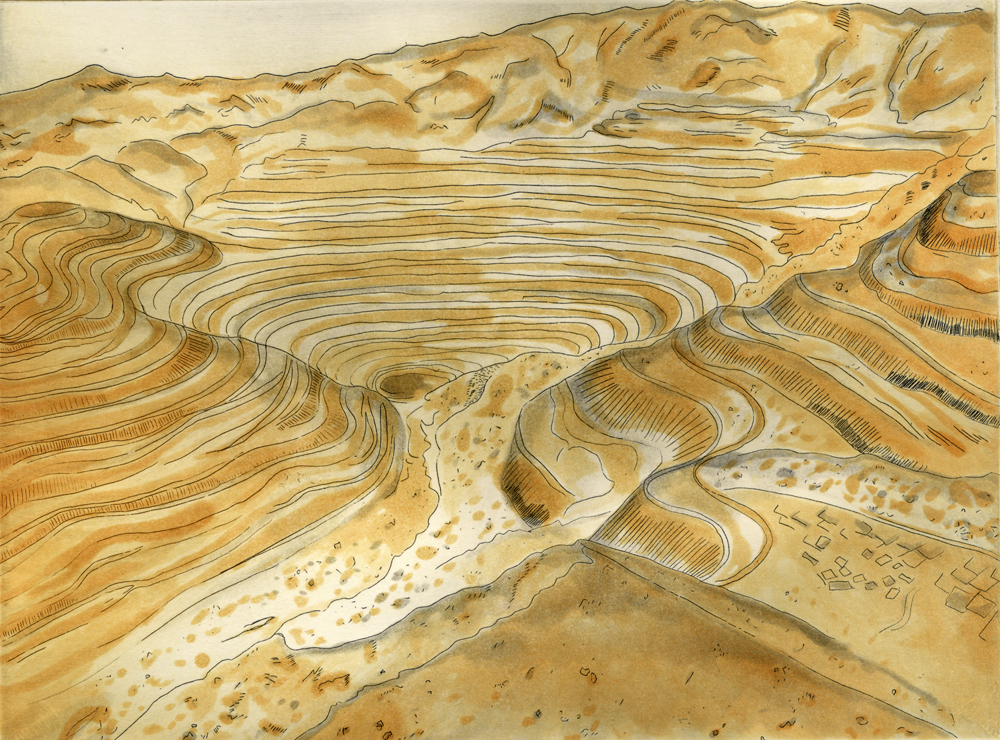Maya Malachowski Bajak, etching

# Extraction: Art on the Edge of the Abyss

Zea Mays Printmaking is excited to participate in this international multimedia, multi-venue, cross-border art intervention that will investigate extractive industry in all of its forms (from mining and drilling to the reckless exploitation of water, soil, trees, marine life, and other natural resources).

While ZMP is continually researching ways to improve, the fact is that printmakers work with materials that are stolen from the earth, e.g., metal plates, pigments, trees, water, and more.

Starting with an Unlikely Materials Exchange for Artists, we will repurpose, plunder, rescue, recycle, trade, and appropriate materials to inspire our community of artists who will create work for local and distant "Green to the Extreme" exhibitions scheduled for 2020-2021. A full-throated response to the challenge of Extractions will include workshops, community engagement and a critical examination of the materials we use to make art.

Stay tuned for more!

 table div table+table+table+table+table+table+table+table+table+table+table+table+table+table+table+table+table+table+table+table+table+table+table+table+table+table+table+table+table+table+table+table div table{width:100%;padding:0}table div table+table+table+table+table+table+table+table+table+table+table+table+table+table+table+table+table+table+table+table+table+table+table+table+table+table+table+table+table+table+table+table div table img{width:96.23%;padding:0;float:none}table div table+table+table+table+table+table+table+table+table+table+table+table+table+table+table+table+table+table+table+table+table+table+table+table+table+table+table+table+table+table+table+table div table td{width:100%;padding:0 1.88% 18px}/* styles */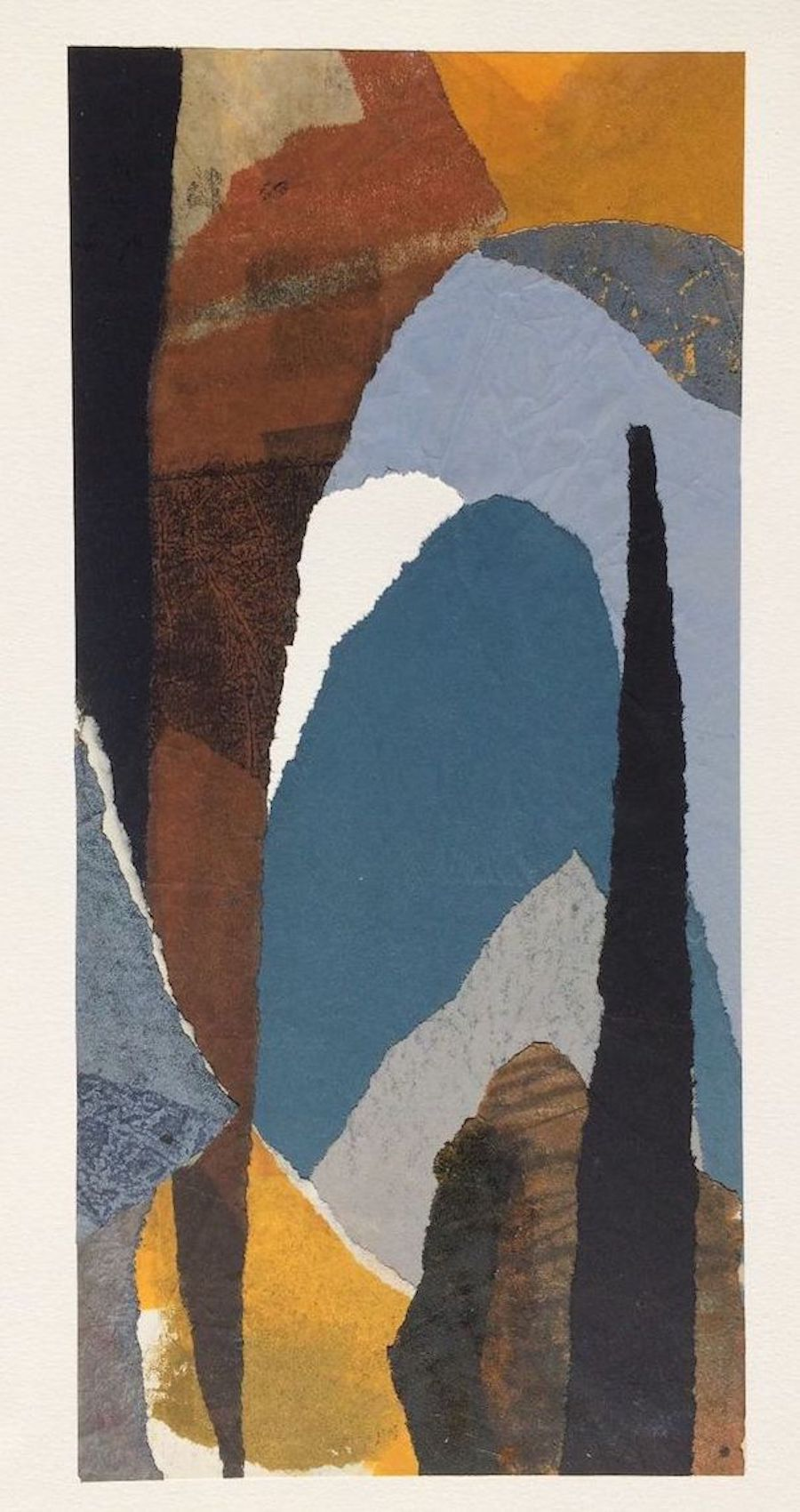Scott McDaniel, collage

# Exhibition: Zea Mays Abstraction

Northampton Senior Center Gallery
October 1-30, 2019
Reception on Arts Night Out, Friday, October 11, 5-7 PM

Curated by Lindsay Berry from the ZMP Flat File Project, including prints by Judith Bowerman, Betsy Feick, Joyce Silverstone, Pamela Crawford, Scott McDaniel, Janet Walerstein Winston, Doris Madsen, Erika Radich, Channing LeFebvre, Paul Lewis and Linda Bills.

Liz Chalfin, Director of Zea Mays Printmaking will present a slide talk about her experiences making prints in Johannesburg, South Africa at Artist Proof Press during the opening reception.

 table div table+table+table+table+table+table+table+table+table+table+table+table+table+table+table+table+table+table+table+table+table+table+table+table+table+table+table+table+table+table+table+table+table+table div table{width:100%;padding:0}table div table+table+table+table+table+table+table+table+table+table+table+table+table+table+table+table+table+table+table+table+table+table+table+table+table+table+table+table+table+table+table+table+table+table div table img{width:96.23%;padding:0;float:none}table div table+table+table+table+table+table+table+table+table+table+table+table+table+table+table+table+table+table+table+table+table+table+table+table+table+table+table+table+table+table+table+table+table+table div table td{width:100%;padding:0 1.88% 18px}/* styles */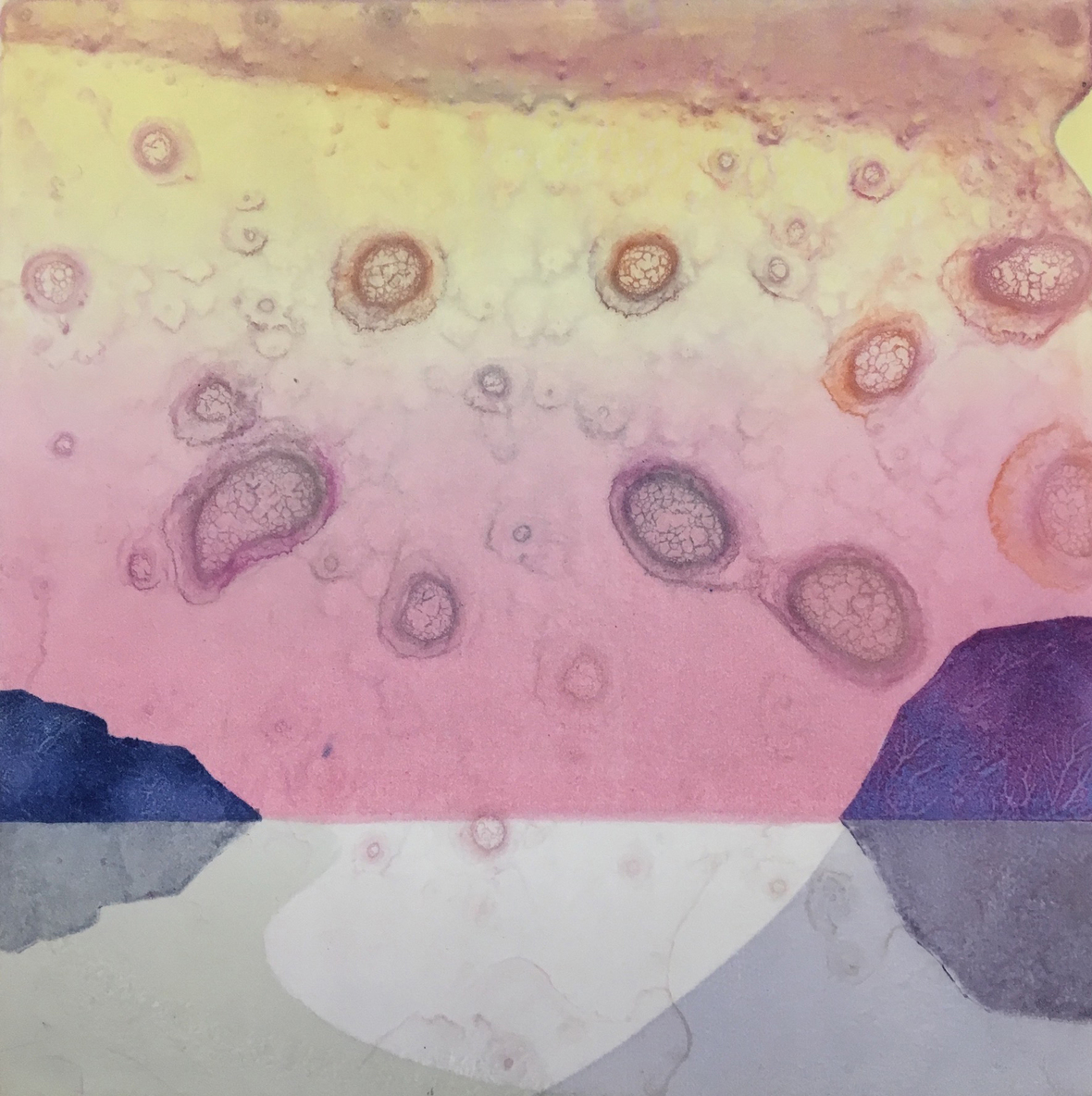Marjorie Morgan, monoprint

# Zea Mays Printmaking Members' Exhibition

## November 7-28, 2019

Easthampton City Arts Gallery

43 Main Street
Easthampton, MA

Reception: Saturday, November 9, 5-8 PM (Easthampton Art Walk)

 table div table+table+table+table+table+table+table+table+table+table+table+table+table+table+table+table+table+table+table+table+table+table+table+table+table+table+table+table+table+table+table+table+table+table+table+table div table{width:100%;padding:0}table div table+table+table+table+table+table+table+table+table+table+table+table+table+table+table+table+table+table+table+table+table+table+table+table+table+table+table+table+table+table+table+table+table+table+table+table div table img{width:96.23%;padding:0;float:none}table div table+table+table+table+table+table+table+table+table+table+table+table+table+table+table+table+table+table+table+table+table+table+table+table+table+table+table+table+table+table+table+table+table+table+table+table div table td{width:100%;padding:0 1.88% 18px}/* styles */## Opportunities and Exhibitions

 table div table+table+table+table+table+table+table+table+table+table+table+table+table+table+table+table+table+table+table+table+table+table+table+table+table+table+table+table+table+table+table+table+table+table+table+table+table+table div table{width:100%;padding:0}table div table+table+table+table+table+table+table+table+table+table+table+table+table+table+table+table+table+table+table+table+table+table+table+table+table+table+table+table+table+table+table+table+table+table+table+table+table+table div table img{width:96.23%;padding:0;float:none}table div table+table+table+table+table+table+table+table+table+table+table+table+table+table+table+table+table+table+table+table+table+table+table+table+table+table+table+table+table+table+table+table+table+table+table+table+table+table div table td{width:100%;padding:0 1.88% 18px}/* styles *//* styles */ New Grounds/Remarque 7th Annual Juried Print Exhibition Event Dates: 12/6/19 - 1/25/20 Entry Deadline: 10/6/19 Massachusetts Cultural Council Artist Fellowship in Printmaking Application Deadline: October 7, 2019 New Studio Printmaker wanted: ABRAZOS PRESS is a nicely appointed 650 foot studio on the ground floor of Miller St. studios with a 30” x 60” Takach Etching press that is used primarily for woodcut, relief, mixed media and pronto plate printmaking. The shop is NOT set up for intaglio printmaking except for drypoint. Flat files and storage are included for each artist. Rent with 24 hour access are \$200.00 per month, with yearly insurance being around \$250, which can be pro-rated. Miller St. is easily accessible to the PORTER SQ RED LINE The studio website is: www.abrazospress.com Please contact: Annie Silverman silverman.annie@gmail.com 617-519-8970 Leonardo Drew: Cycles from the Collections of Jordan D. Schnitzer and His Family Foundation- University Art Museum, UMass, Amherst. September 20-December 8, 2019. Reception for the Artist: September 19, 4-7:30 PM with a Panel Discussion 4:30-5:30 PM.
 table div table+table+table+table+table+table+table+table+table+table+table+table+table+table+table+table+table+table+table+table+table+table+table+table+table+table+table+table+table+table+table+table+table+table+table+table+table+table+table+table div table{width:100%;padding:0}table div table+table+table+table+table+table+table+table+table+table+table+table+table+table+table+table+table+table+table+table+table+table+table+table+table+table+table+table+table+table+table+table+table+table+table+table+table+table+table+table div table img{width:96.23%;padding:0;float:none}table div table+table+table+table+table+table+table+table+table+table+table+table+table+table+table+table+table+table+table+table+table+table+table+table+table+table+table+table+table+table+table+table+table+table+table+table+table+table+table+table div table td{width:100%;padding:0 1.88% 18px}/* styles */## Member News

 table div table+table+table+table+table+table+table+table+table+table+table+table+table+table+table+table+table+table+table+table+table+table+table+table+table+table+table+table+table+table+table+table+table+table+table+table+table+table+table+table+table+table+table div table{width:100%;padding:0}table div table+table+table+table+table+table+table+table+table+table+table+table+table+table+table+table+table+table+table+table+table+table+table+table+table+table+table+table+table+table+table+table+table+table+table+table+table+table+table+table+table+table+table div table img{width:96.23%;padding:0;float:none}table div table+table+table+table+table+table+table+table+table+table+table+table+table+table+table+table+table+table+table+table+table+table+table+table+table+table+table+table+table+table+table+table+table+table+table+table+table+table+table+table+table+table+table div table td{width:100%;padding:0 1.88% 18px}/* styles */table div table+table+table+table+table+table+table+table+table+table+table+table+table+table+table+table+table+table+table+table+table+table+table+table+table+table+table+table+table+table+table+table+table+table+table+table+table+table+table+table+table+table+table+table+table+table div table{width:100%;padding:0}table div table+table+table+table+table+table+table+table+table+table+table+table+table+table+table+table+table+table+table+table+table+table+table+table+table+table+table+table+table+table+table+table+table+table+table+table+table+table+table+table+table+table+table+table+table+table div table img{width:96.23%;padding:0;float:none}table div table+table+table+table+table+table+table+table+table+table+table+table+table+table+table+table+table+table+table+table+table+table+table+table+table+table+table+table+table+table+table+table+table+table+table+table+table+table+table+table+table+table+table+table+table+table div table td{width:100%;padding:0 1.88% 18px}/* styles */